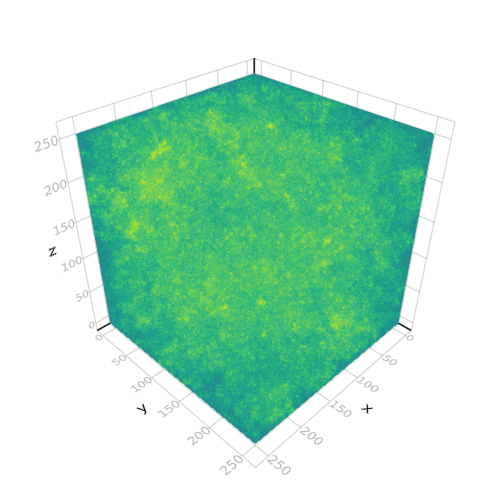## Coloured fractional Brownian noise field

```
using FFTW

using AbstractPlotting

"This example was contributed by Harmen Stoppels (@haampie)"

# Obtain approximately fractal Brownian noise, appropriately damping
# the high frequencies of Fourier transformed spatial white noise,
# and (inverse) Fourier transforming the result back into the spatial domain.
function cloud(n = 256, p = 0.75f0)
ωs = fft(randn(Float32, n, n, n))
r = Float32[0:n÷2; n÷2-1:-1:(iseven(n) ? 1 : 0)]
xs, ys, zs = reshape(r, :, 1, 1), reshape(r, 1, :, 1), reshape(r, 1, 1, :)
ωs ./= (1.0f0 .+ (xs.^2 .+ ys.^2 .+ zs.^2) .^ p)
return real.(ifft(ωs))
end

z = cloud(256, 0.75)

volume(z; algorithm = :mip, colorrange = extrema(z))

```